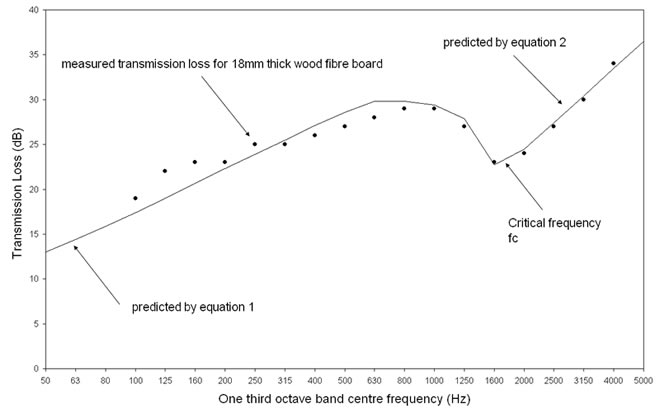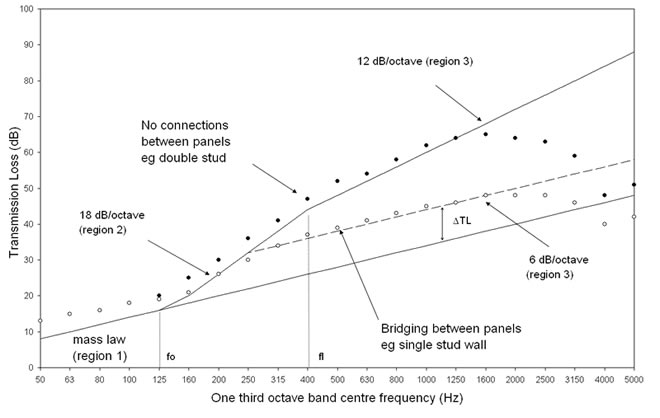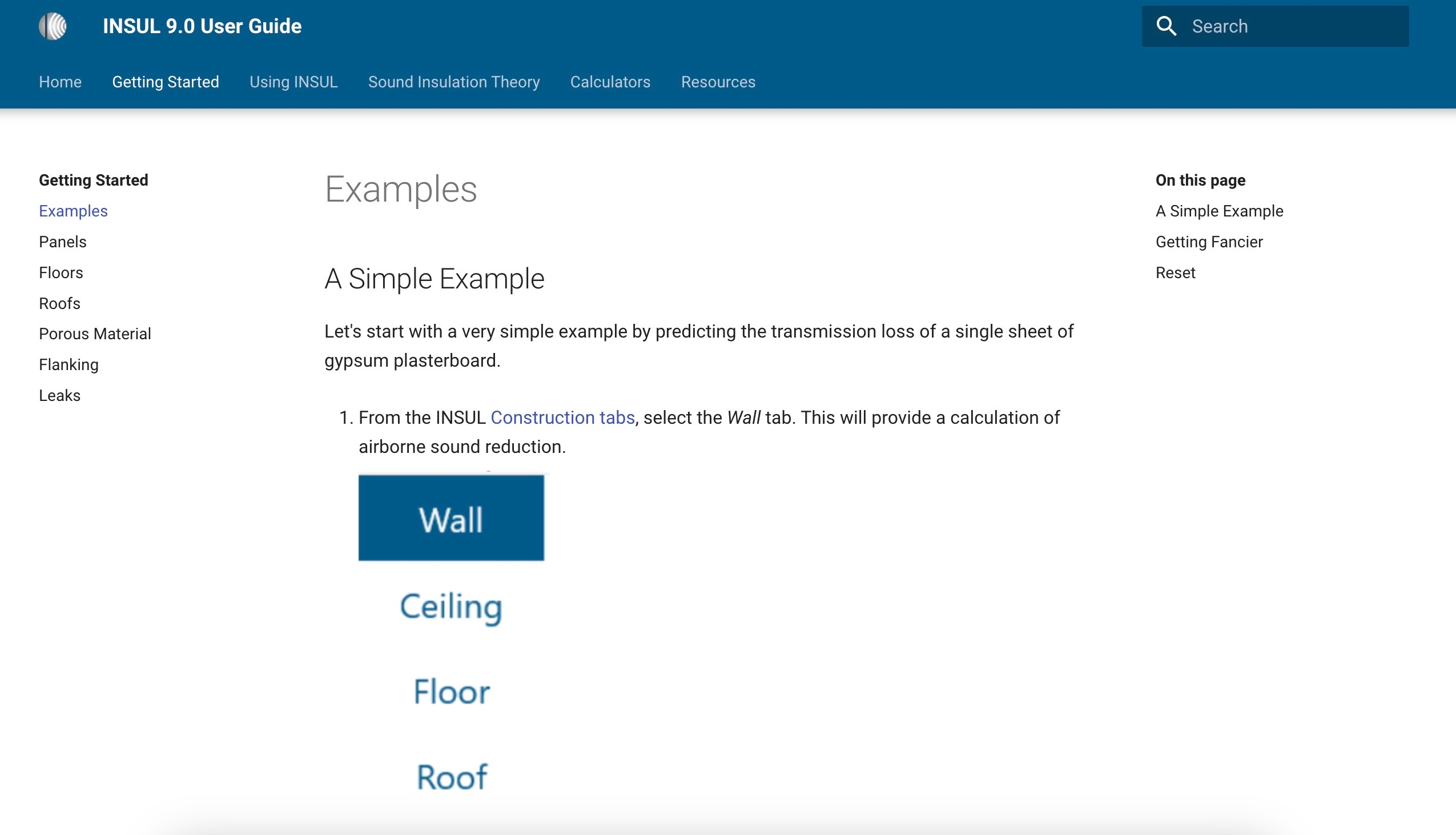## Single Panels

The sound transmission through a single panel can be approximated to a good degree of accuracy using only the mass per unit area (m) and the Modulus of Elasticity (E) of the panel.

At low and mid frequencies the transmission loss (TL or R) is calculated from the well known mass law equation:

TL = 20 log ( mf ) - 48

where f is the frequency (Hz).

At higher frequencies the coincidence effect reduces the sound insulation and the transmission loss is given by:

TL = 20 log ( mf ) + 10 log ( 2fη / πfc ) + 20 log ( π / ρc )

Where fc is the critical frequency (which can be calculated from E), η is the loss factor, ρ is the air density and c is the speed of sound.

For thick heavy panels such as Brick or Concrete additional transmission takes place due to shear waves and the TL at high frequencies is reduced. INSUL takes this effect into account.

## Low frequency TL

At low frequencies the radiation efficiency of a finite sized partition is reduced and the measured transmission loss is greater than the simple mass law. This effect is more pronounced for elements such as windows which are often tested with small areas. However even for normal tests carried out to ISO 140 with an area of 10-12m² the effect is significant at the lowest test frequencies. INSUL can take account of this effect.

A further effect that must be accounted for are the panel modes for light weight timber or steel framed walls.  This is especially important for stud spacings of 400mm (16") or less.  The STC rating is especially sensitive to this as the first panel mode can often fall in or very close to the 125 Hz 1/3 octave band, and the STC rating can be determined completely by this band due to the 8 dB rule.  Reductions of 10 to 13 dB in STC rating can occur by changing stud spacings from 24" to 16".## Double panels

INSUL predicts the transmission loss of double panel systems in 4 different frequency regions.

Region 1
At low frequencies the transmission loss is determined primarily by the mass law. The TL increases at 6 dB/octave but INSUL can account for the inefficient radiation of low frequencies (link to section on single panels).

Region 2
Above the mass-air-mass resonance frequency of the partition (fo) determined by the mass of the panels and the air gap, the TL increases at 18 dB/octave as the two sides become decoupled.

Region 3
When the cavity width becomes comparable to a wavelength at frequency fl the cavity modes couple the panels together and the TL increases at 12 dB/octave.

Region 4
Solid connections act as sound bridges between the two panels and the TL is limited to a constant amount above the mass law, and increases at only 6 dB/octave

## References

If you are interested in reading more about the theoretical background that INSUL is based on, the following references provide an excellent introduction.

1. B.H.Sharp, Prediction Methods for the Sound Transmission of Building Elements. Noise Control Engineering Vol 11 1978

2. L.Cremer M.Heckel E.E.Ungar, Structureborne Sound (Springer Verlag,1988)

3. F.Fahy, Sound and Structural Vibration (Academic Press, 1985)

4. J.H. Rindel, Sound Radiation form Building Structures and Acoustical Properties of Thick Plates. COMETT-SAVOIR Course Notes, CSTB Grenoble## User Guide

The INSUL version 9.0 User Guide includes advice on getting started with INSUL modelling.  It also contains information about the technical foundations of INSUL which can assist with developing robust models of sound insulation performance.

The INSUL version 9.0 User Guide is accessible here.